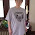## Saturday, October 31, 2015

### 96 47 110 | The Death of Al Molinaro of Happy Days

Dying at age 96 stands out.

Happy = 8+1+16+16+25 = 66
Days = 4+1+25+19 = 49
Happy Days = 115

Happy = 8+1+7+7+7 = 30
Days = 4+1+7+1 = 13/22
Happy Days = 43/22

Big = 2+9+7 = 18
Al = 1+12 = 13
Delvecchio = 4+5+12+22+5+3+3+8+9+15 = 86
Big Al Delvecchio = 117

Big = 2+9+7 = 18
Al = 1+3 = 4
Delvecchio = 4+5+3+4+5+3+3+8+9+6 = 50
Big Al Delvecchio = 72

Notice he died in Glendale, California.

Glendale = 7+12+5+14+4+1+12+5 = 60
California = 3+1+12+9+6+15+18+14+9+1 = 88
Glendale, California = 148

Glendale = 7+3+5+5+4+1+3+5 = 33
California = 3+1+3+9+6+6+9+5+9+1 = 52
Glendale, California = 85

http://www.cnn.com/2015/10/31/entertainment/happy-days-star-al-molinaro-dies/index.html

Albert = 1+12+2+5+18+20 = 58 (Al = 1+12 = 13)
Francis = 6+18+1+14+3+9+19 = 70
Molinaro = 13+15+12+9+14+1+18+15 = 97
Albert Francis Molinaro = 225
Al Molinaro = 110

Albert = 1+3+2+5+9+2 = 22 (Al = 1+3 = 4)
Francis = 6+9+1+5+3+9+1 = 34/43
Molinaro = 4+6+3+9+5+1+9+6 = 43
Albert Francis Molinaro = 99/108
Al Molinaro = 47

6/24/1919 = 6+24+19+19 = 68
6/24/1919 = 6+24+(1+9+1+9) = 50
6/24/1919 = 6+2+4+1+9+1+9 = 32
6/24/19 = 6+24+19 = 49

10/30/2015 = 10+30+20+15 = 75
10/30/2015 = 10+30+(2+0+1+5) = 48
10/30/2015 = 1+0+3+0+2+0+1+5 = 12
10/30/15 = 10+30+15 = 55

http://www.cnn.com/2015/10/31/entertainment/happy-days-star-al-molinaro-dies/index.html

1.I think I just found something awesome. I was looking at the "Secret Space Program" youtube channel (they're having a convention this weekend" and I noticed that they had subscribed to another youtube channel so I went there a found this..
Shuttle Challenger:Flat Earth Misdirection
If this is true it's bombshell! Check it out it's only 1:58 minutes long.

1.Here's the original video....
1986 Challenger space shuttle disaster crew still alive ?

2.I've seen it, I'm not that into the "this person looks like this person" thing. Look at the videos on YouTube made about me asserting I am someone else. People look at those and think it is convincing, even though it is ridiculous. That said, I think it could be entirely possible and would make a lot of sense in regards to what is presented in the video.

2.I think a couple of them look a lot alike. The pictures could be doctored but I'm going to decode the Challenger mission anyway to see what comes up. With NASA you can never tell could be true. Thanks

1.http://freetofindtruth.blogspot.com/2014/08/33-nasa-challenger-disaster-hoax-and.html

This is an older blog post on the "disaster".